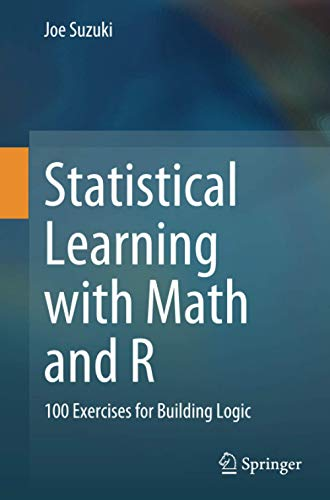# Statistical Learning with Math and R: 100 Exercises for Building Logic

• Length: 232 pages
• Edition: 1
• Publisher:
• Publication Date: 2020-10-20
• ISBN-10: 9811575673
• ISBN-13: 9789811575679
2.0
Description

The most crucial ability for machine learning and data science is mathematical logic for grasping their essence rather than knowledge and experience. This textbook approaches the essence of machine learning and data science by considering math problems and building R programs.

As the preliminary part, Chapter 1 provides a concise introduction to linear algebra, which will help novices read further to the following main chapters. Those succeeding chapters present essential topics in statistical learning: linear regression, classification, resampling, information criteria, regularization, nonlinear regression, decision trees, support vector machines, and unsupervised learning.

Each chapter mathematically formulates and solves machine learning problems and builds the programs. The body of a chapter is accompanied by proofs and programs in an appendix, with exercises at the end of the chapter. Because the book is carefully organized to provide the solutions to the exercises in each chapter, readers can solve the total of 100 exercises by simply following the contents of each chapter.

This textbook is suitable for an undergraduate or graduate course consisting of about 12 lectures. Written in an easy-to-follow and self-contained style, this book will also be perfect material for independent learning.